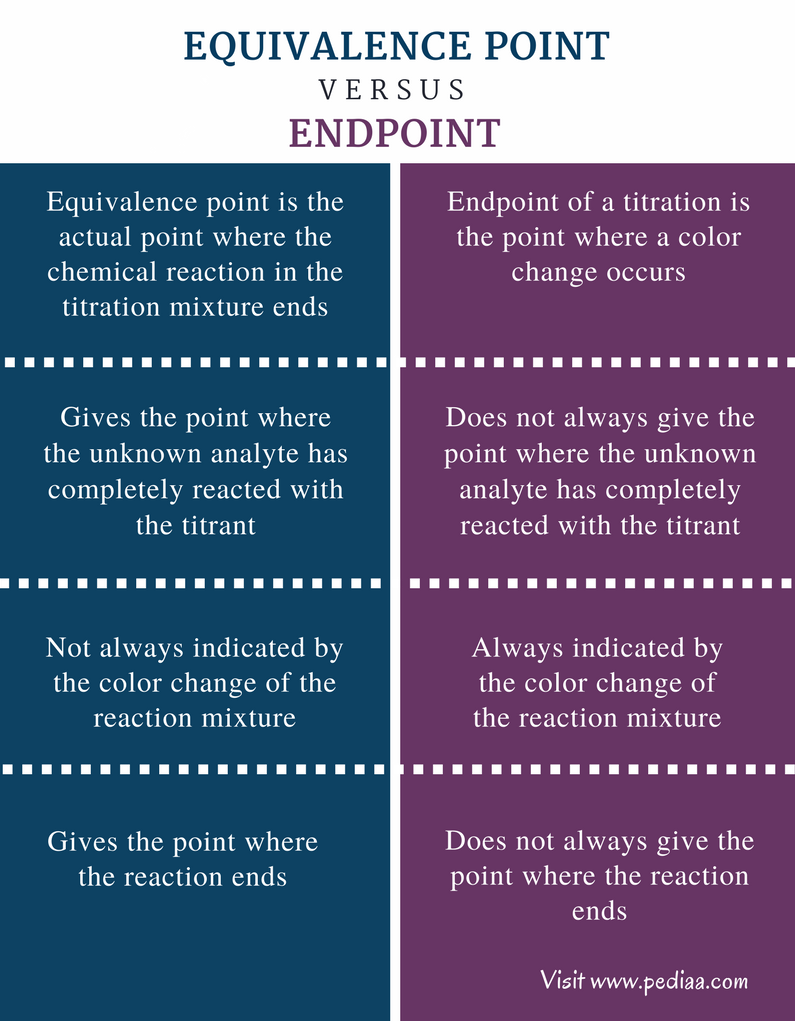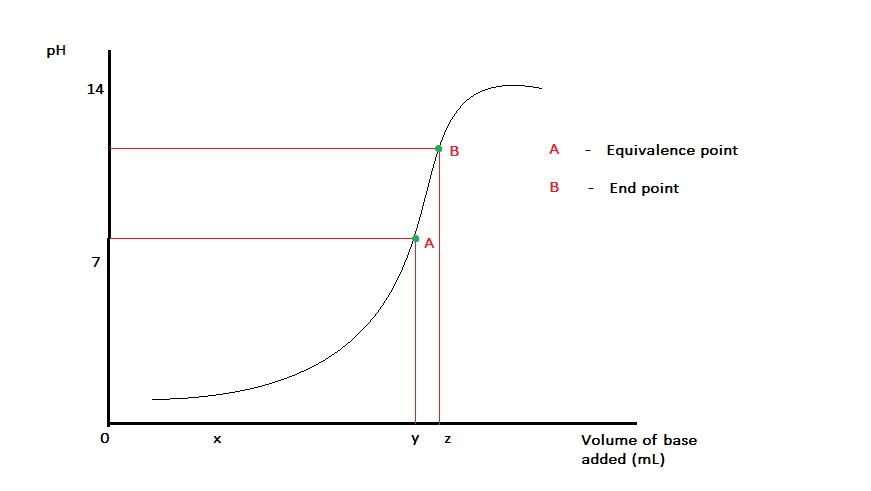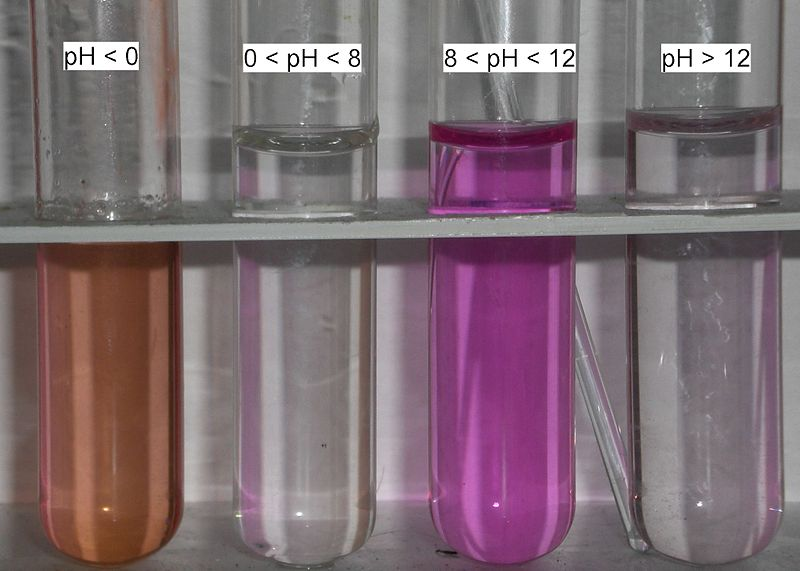# Difference Between Equivalence Point and Endpoint

## Main Difference – Equivalence Point vs Endpoint

Titration methods are often used to identify and quantify the components in a solution mixture. Some titrations are done along with an indicator that is helpful in indicating the end of the chemical reaction. This indication is given by changing the color of the system. But some reactants act as indicators themselves. Thus, indicators are not used in all systems. The results of a titration mainly depend on the person who does the titration since different people identify the endpoint of a titration at different points. However, the end point is not the point where the reaction actually ends. The end of the reaction is given by the equivalence point. The endpoint indicates that the equivalence point has been reached. The main difference between equivalence point and endpoint is that equivalence point is the actual point where the chemical reaction ends whereas end point is the point where the color change occurs in the system.

### Key Areas Covered

1. What is Equivalence Point
– Definition, Properties, Examples
2. What is Endpoint
– Definition, Properties, Example
3. What is the Difference Between Equivalence Point and Endpoint
– Comparison of Key Differences

Key terms: Endpoint, Equivalence Point, Indicator, Molarity, Phenolphthalein, Titration## What is Equivalence Point

Equivalence Point is the actual point where the chemical reaction in a titration mixture ends. A titration is done often to determine the concentration of a substance in a liquid. If the substance is known, we can use a titrant (a solution used to determine the concentration of a component in a liquid mixture) with a known concentration that can react with the substance. The titrant is called a standard solution because its exact molarity is known.

For example, let us consider the reaction between NaOH and HCl. This is an acid-base reaction. We can use either NaOH or HCl as the titrant of the concentration. The titrant is placed in the burette and is added slowly to the titrand/analyte until a color change occurs in the reaction mixture. An indicator should be used as NaOH or HCl are not self-indicators.  The point where a color change occurs is taken as the endpoint of the titration. But it is not the equivalence point of the reaction.

Here, the equivalence point is the point where all HCl molecules have reacted with NaOH (or the point where all NaOH molecules have reacted with HCl). Here, the moles of titrant should be equal to the moles of the unknown analyte.Figure 1: Titration curve for a titration of an acid with a base

### Methods of Determining the Equivalence Point

• Color change of self-indicators – In reactions involving self-indicators as reactants, the color change indicates the equivalence point of the titration since indicators are not used.
• Endpoint – Sometimes, equivalence point can be considered as the endpoint since they are approximately equal.
• Conductance – Conductance can also be used to determine the equivalence point of the titration. Here, the conductance should be measured throughout the titration, and the equivalence point is where a rapid change of conductance occurs. This is a bit difficult method.
• Spectroscopy – This method can be used for colorful reaction mixtures. The determination is done according to the rapid change in wavelengths that are absorbed by the sample.

## What is Endpoint

The endpoint of a titration is the point where a color change occurs. Normally, acids and bases are colorless solutions. Therefore, to determine the end of the neutralization reaction of an acid with a base, an indicator that is able to change the color of the reaction mixture with changes in pH is used. For example, Phenolphthalein is a common indicator used in acid base reactions. The color change of this indicator is given at 8.3 (colorless) and 10.0 (pink). But this indicator is not suitable for reactions that occur at low pH values. Therefore, the indicator should be chosen accordingly.Figure 2: Color of phenolphthalein at different pH values

The endpoint is not the point where the total amount of the unknown has completely reacted with the titrant. In reactions where self-indicators are involved, the end point is given when the whole amount of the unknown has reacted with titrant. Therefore, the endpoint is equal to the equivalence point here. For example, the half-reaction of conversion of Cr2O72- to C3+ changes the color of the medium from orange to green. This is because the color of Cr (+6) in aqueous solution is orange and the color of Cr (+3) in the aqueous solution is green. Therefore, this color change is taken as the endpoint of that reaction.

## Difference Between Equivalence Point and Endpoint

### Definition

Equivalence Point: Equivalence point is the actual point where the chemical reaction in the titration mixture ends.

Endpoint: The endpoint of a titration is the point where a color change occurs.

### Stoichiometry

Equivalence Point: Equivalence point gives the point where the unknown analyte has completely reacted with the titrant.

Endpoint: Endpoint does not always give the point where the unknown analyte has completely reacted with the titrant.

### Color Change

Equivalence Point: The color change of the reaction mixture does not always indicate the exact equivalence point.

Endpoint: The color change always indicates the endpoint.

### End of Reaction

Equivalence Point: Equivalence point gives the point where the reaction ends.

Endpoint: Endpoint does not always give only the end of the reaction.

### Conclusion

Although the endpoint is normally considered as the equivalence point, they are not the same. But since there is only a slight difference between equivalent point and end point and it can be considered as the same for laboratory practices. The endpoint always comes after the equivalence point. The main difference between Equivalence Point and Endpoint is that equivalence point is the end of the reaction whereas end point is the point where a color change occurs.

##### References:

1.”Titration Fundamentals.” Chemistry LibreTexts. Libretexts, 21 July 2016. Web. Available here. 12 July 2017.
2.Helmenstine, Anne Marie. “Here’s What the Equivalence Point Means in Chemistry.” ThoughtCo. N.p., n.d. Web. Available here. 12 July 2017.

##### Image Courtesy:

1. “Phenolphtalein” By User:Siegert – Own work (Public Domain) via Commons Wikimedia